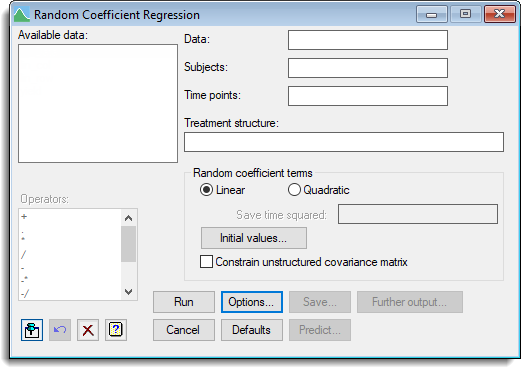1. Home
2. Random Coefficient Regression

# Random Coefficient Regression

Select menu: Stats | Mixed Models (REML) | Random Coefficient Regression

Random coefficient regression seeks to model individual profiles over time using linear models with common parameters within treatment groups, enabling for random variation about these parameters for individuals.

Stats | Mixed Models (REML) | Random Coefficient Regression.
2. Fill in the fields as required then click Run.

You can set additional Options then after running, you can save the results by clicking Save.## Available data

This lists data structures appropriate to the current input field. The contents will change as you move from one field to the next. Double-click on a name to copy it to the current input field or type the name.

## Data

Specifies the response variate (dependent variate).

## Subjects

Specifies the subjects or individuals.

## Time points

Specifies the time points for the observations for the subjects.

## Treatment structure

The imposed treatment factors and covariates for which the effect of specified levels or values are of interest. The model is described using a formula, which can combine main effects and interactions of factors and also covariates.

## Random coefficient terms

Lets you select the terms to use to model the profiles over time. You can specify inear or linear plus quadratic. If you specify linear plus quadratic then you can specify the name of identifier to save the time squared values within using the Save time squared field.

 Initial values Opens a dialog that can be used to specify the initial values for the covariance matrix across the random effect terms Constrain unstructured covariance matrix When selected, the covariance structure is constrained to be positive-definite. By default, this constraint is relaxed using an unstructured covariance matrix to describe the covariance across the random effect terms.

## Operators

This provides a quick way of entering operators in the treatment structure formula. Double-click on the required symbol to copy it to the current input field. You can also type in operators directly. You can also type in operators directly. See Operators in model formula for a description of each.